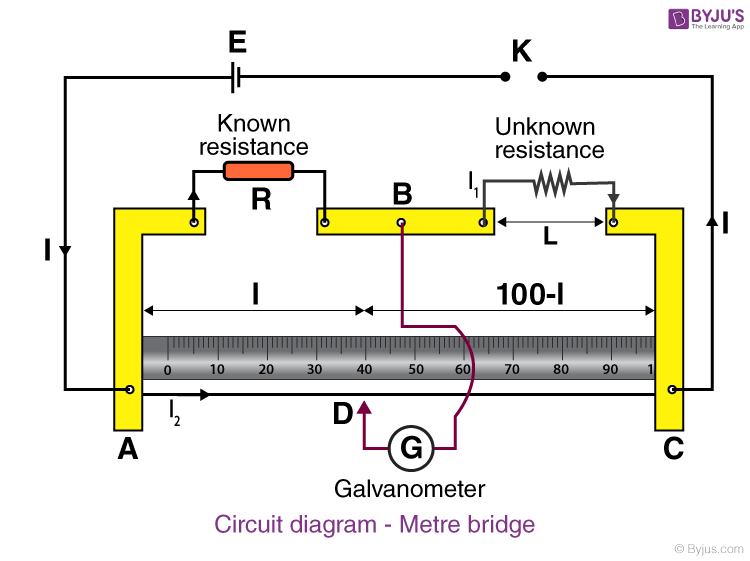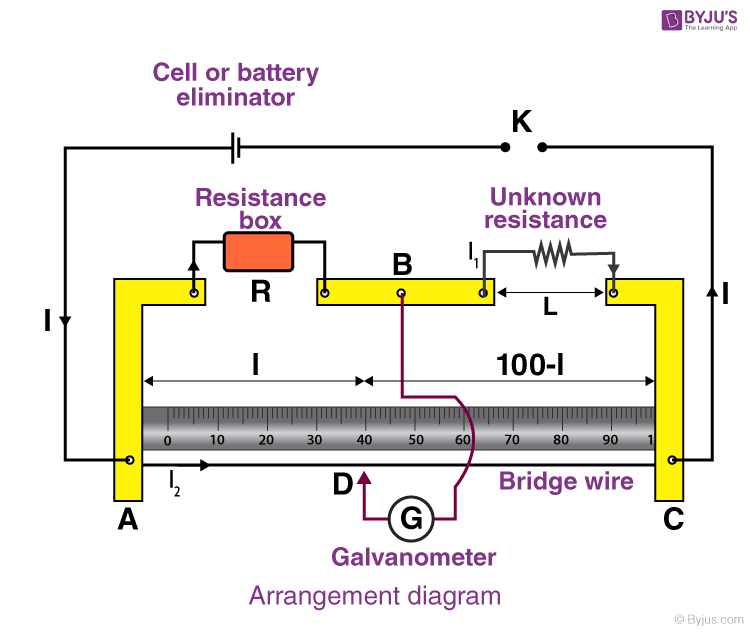# To find resistance of a given wire using metre bridge and to determine the resistivity of its material

A metre bridge, also known as slide wire bridge is an instrument that works on the principle of Wheatstone bridge. It is used to determine the unknown resistance of a conductor. Below is an experiment on how to find the resistance of a given wire using metre bridge and to determine the resistivity of its material.

## Aim

To find resistance of a given wire using metre bridge and hence determine the resistivity (specific resistance) of its material.

## Materials Required

1. A metre bridge
2. A Leclanche cell (battery eliminator)
3. A galvanometer
4. A resistance box
5. A jockey
6. A one-way key
7. A resistance wire
8. A screw gauge
9. A metre scale
10. A set square
11. Connecting wires
12. A piece of sandpaper

## Theory

Metre bridge apparatus is also known as a slide wire bridge. It is fixed on the wooden block and consists of a long wire with a uniform cross-sectional area. It has two gaps formed using thick metal strips to make the Wheatstone’s bridge.

Then according to Wheatstone’s principle, we have:

$\frac{X}{R}=\frac{l}{(100-l)}$

The unknown resistance can be calculated as:

$X=R\frac{l}{(100-l)}$

Then the specific resistance of the material of the is calculated as:

$\rho =\frac{\pi r^{2}X}{L}$

Where,

• L is the length of the wire
• r is the radius of the wire

## Circuit Diagram## Procedure

1. The arrangement of the apparatus should be as shown in the circuit diagram.
2. The wire whose resistance is to be determined should be connected in the right gap between C and B without any formation of loops.
3. The resistance box should be connected in the left gap between A and B.
4. All the other connections should be as shown in the circuit diagram.
5. Plug the key K in place of 2-ohm resistance in the resistance box.
6. The jockey should be first touched gently to the left end and then to the right end of the bridge.
7. The deflections in the galvanometer should be in opposite directions and if it is in one direction then the circuit connections are not correct. Note the galvanometer deflection.
8. Let D be the null point where the jockey is touching the wire. The movement of the jokey should be gentle from left to the right of the galvanometer.
9. Take a 12 value from the resistance box should be taken such that when the jockey is nearly in the middle of the wire, there shouldn’t be any deflection.
10. Note the position of D to know the length of AD = l.
11. Four sets of observations should be taken by changing the value of 12.
12. Record the observations in a tabular form.
13. Stretch the resistance wire to find its length using a metre scale.
14. Using screw gauge measure the diameter of the wire at four different places keeping it in a mutually perpendicular direction.
15. Record the observations in the table.## Observations

Length of given wire L =…….cm

Table for unknown resistance (X)

 Resistance from box, R (Ohm) Length AB = l (cm) Length BC = (100-l) (cm) Unknown Resistance X = [R(100-l)]/L (Ohm) 0.5 58.3 41.7 0.35 0.7 60.7 39.3 0.45 1 61.9 38.1 0.61 1.5 61.1 38.9 0.95

Least count of the screw gauge

Pitch of screw gauge = …….mm

Total no.of divisions on the circular scale = ………..

∴ L.C of the given screw gauge = $\frac{pitch}{no.\,of\,divisions\,on\,the\,circular\,scale}$ = ……mm

Zero error e = ……mm

Zero correction c = -e = ……mm

 Main scale reading (mm) Circular scale reading Total reading (diameter) (mm) Mean D (mm) Mean radius (D/2) (mm) 0 43 0.43 0.42 0.21

## Calculations

Calculation for X

The value of l is determined from the position of D and recorded in column 3 of table 1.

Find length (100 – l) cm and write in column 4.

Calculate X and write in column 5,

$Mean X = \frac{X_{1}+X_{2}+X_{3}+X_{4}}{4}=……ohm$

Calculation for D

Mean corrected diameter = $\frac{D_{1}(a)+D_{1}(b)+…..+D_{4}(a)+D_{4}(b)}{8}=……mm\,=….cm$

Calculation for specific resistance

Specific resistance of the material of the given wire,

$\rho =X.\frac{\pi D^{2}}{4L}=…..ohm\,cm=……ohm\,m$

Standard value of the specific resistance of the material of the given wire,

ρ0 = …..ohm.m

Percentage error = $\frac{\rho -\rho _{0}}{\rho _{0}}×100$ = ………%

## Result

1. The value of unknown resistance X = …….
2. The specific resistance of the material of the given wire = ………
3. Percentage error = …….

## Precautions

1. The connections should be neat, tight and clean.
2. Plugs should be tightly connected in the resistance box.
3. The movement of the jockey should be gentle and it shouldn’t be rubbed.
4. The key K should be inserted only when the observations are to be taken.
5. The null point should be between 45cm and 55cm.
6. To avoid the error of parallax, the set square should be used to note the null point.
7. There shouldn’t be any loops in the wire.
8. The diameter of the wire should be measured in two perpendicular directions that are mutual.

## Sources Of Error

1. The screws of the instrument might be loose.
2. The wire might be of non-uniform diameter.
3. There might be backlash error in the screw gauge.

## Viva Questions

Q1. Why is metre bridge called so?

Ans: It is called metre bridge because the bridges use one-meter long wire.

Q2. What is null point?

Ans: Null point is defined as the point at which galvanometer reads 0 deflections.

Q3. Why is bridge method better than Ohms law of measurement?

Ans: Bridge method is better than the Ohms law of measurement because of the null method.

Q4. What is the range of measurement of resistance using a Wheatstone bridge?

Ans: The range of measurement of resistance using a Wheatstone bridge is between 1Ω to a few megaohms.

Q5. How can a Wheatstone bridge be used for the measurement of physical parameters?

Ans: Wheatstone bridge is used to measure the physical parameters like temperature, light, etc using an operational amplifier and rectifiers are used for the conversion of A.C to D.C.